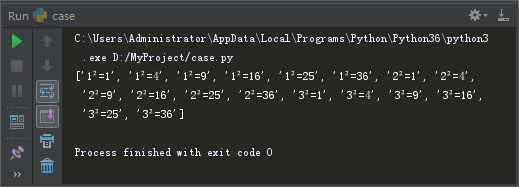﻿ Python3萌新入门笔记（22） – 魔力Python

# Python3萌新入门笔记（22）

Python教程 6334浏览

```lst = [x * x for x in range(1, 7)]  # 列表推导式：循环获取范围1~6的整数，计算乘积后添加到新列表。
print(lst)  # 显示输出结果为：[1, 4, 9, 16, 25, 36]```

```lst = [x * x for x in range(1, 7) if x % 2 == 0]  # 列表推导式：循环获取范围1~6的整数，符合条件时，计算乘积后添加到新列表。
print(lst)  # 显示输出结果为：[4, 16, 36]```

```number = [1, 2, 3, 4, 5, 6]  # 整数列表
square = [1, 4, 9, 16, 25, 36]  # 平方列表
lst = ['{0}²={1}'.format(str(x), str(y)) for x in number for y in square if x <= 3]
print(lst)``````number = [1, 2, 3, 4, 5, 6]  # 整数列表
square = [1, 4, 9, 16, 25, 36]  # 平方列表
lst = ['{0}²={1}'.format(str(x), str(y)) for x in number for y in square if x <= 3 and x * x == y]
print(lst) # 显示输出结果为：['1²=1', '2²=4', '3²=9']```

```number = [1, 2, 3, 4, 5, 6]
square = [1, 4, 9, 16, 25, 36]
lst = [] # 此部分用列表推导式替代
for x in number: # 此部分用列表推导式替代
for y in square: # 此部分用列表推导式替代
if x <= 3 and x * x == y: # 此部分用列表推导式替代
lst.append('{0}²={1}'.format(str(x), str(y))) # 此部分用列表推导式替代
print(lst)```

lambda表达式的官方定义：一个匿名内联函数，由一个表达式组成，在函数被调用时求值。

lambda表达式可用于函数的参数。

```def iseven(n):  # 定义验证数字是否偶数的函数
if n % 2 == 0:  # 判断参数是否为偶数
return True  # 符合条件返回真值
else:
return False #否则返回假值

lst = list(filter(iseven, number)) # 通过函数iseven对每个number的元素进行验证，验证为真的保留，并将最终结果转换为list。
print(lst) # 显示输出结果为：[2, 4, 6]```

filter函数会把number的每一个元素作为函数iseven的参数传入，进行计算，并将返回结果。

```number = [1, 2, 3, 4, 5, 6]  # 整数列表

lst = list(filter(lambda x: x % 2 == 0, number)) # 通过lambda表达式对每个number的元素进行验证，并将所有验证结果转换为list。
print(lst) # 显示输出结果为：[2, 4, 6]```

1、列表推导式；

2、lambda表达式。

1、square：平方

2、even：偶数

3、comprehension：理解

4、lambda：希腊字母λ的英文名称，大名鼎鼎的游戏半条命里面的logo就是这个。name_set = {‘邢佳栋’, ‘李学庆’, ‘高昊’, ‘潘粤明’, ‘戴军’, ‘薛之谦’, ‘贾宏声’, ‘于波’, ‘李连杰’, ‘王斑’, ‘蓝雨’, ‘刘恩佑’,’任泉’, ‘李光洁’, ‘姜文’, ‘黑龙’, ‘张殿菲’, ‘邓超’, ‘张杰’, ‘杨坤’, ‘沙溢’, ‘李茂’, ‘黄磊’, ‘于小伟’, ‘刘冠翔’,’秦俊杰’, ‘张琳’, ‘陈坤’, ‘黄觉’, ‘邵峰’, ‘陈旭’, ‘马天宇’, ‘杨子’, ‘邓安奇’, ‘赵鸿飞’, ‘马可’, ‘黄海波’,’黄志忠’, ‘李晨’, ‘后弦’, ‘王挺’, ‘何炅’, ‘朱亚文’, ‘胡军’, ‘许亚军’, ‘张涵予’, ‘贾乃亮’, ‘陆虎’, ‘印小天’,’于和伟’, ‘田亮’, ‘夏雨’, ‘李亚鹏’, ‘胡兵’, ‘王睿’, ‘保剑锋’, ‘于震’, ‘苏醒’, ‘胡夏’, ‘张丰毅’, ‘刘翔’,’李玉刚’, ‘林依轮’, ‘袁弘’, ‘朱雨辰’, ‘丁志诚’, ‘黄征’, ‘张子健’, ‘许嵩’}

### 网友最新评论 (11)

1. ```name_set = {'邢佳栋', '李学庆', '高昊', '潘粤明', '戴军', '薛之谦', '贾宏声', '于波', '李连杰', '王斑', '蓝雨', '刘恩佑', '任泉', '李光洁', '姜文', '黑龙', '张殿菲', '邓超', '张杰', '杨坤', '沙溢', '李茂', '黄磊', '于小伟', '刘冠翔', '秦俊杰', '张琳', '陈坤', '黄觉', '邵峰', '陈旭', '马天宇', '杨子', '邓安奇', '赵鸿飞', '马可', '黄海波', '黄志忠', '李晨', '后弦', '王挺', '何炅', '朱亚文', '胡军', '许亚军', '张涵予', '贾乃亮', '陆虎', '印小天', '于和伟', '田亮', '夏雨', '李亚鹏', '胡兵', '王睿', '保剑锋', '于震', '苏醒', '胡夏', '张丰毅', '刘翔', '李玉刚', '林依轮', '袁弘', '朱雨辰', '丁志诚', '黄征', '张子健', '许嵩'}
lst1 = [x for x in name_set if '李' in x]  # 使用列表推导式获取新的列表。
lst2 = list(filter(lambda x: x == '李', name_set))  # 使用lambda表达式进行筛选，将结果转换为列表。
print(lst1)  # 显示输出结果为：['李光洁', '李玉刚', '李学庆', '李茂', '李连杰', '李亚鹏', '李晨']
print(lst2)  # 显示输出结果为：['李光洁', '李玉刚', '李学庆', '李茂', '李连杰', '李亚鹏', '李晨']
```
小楼一夜听春语5年前 (2017-09-13)回复
2. 已阅，写的很好。 😆
走路爱走神4年前 (2018-05-25)回复
3. 文中“filter函数会把number的每一个元素作为函数iseven的参数传入，进行计算，并将返回结"句子不完整。
alfred34年前 (2018-06-09)回复
• 已修正，谢谢！
小楼一夜听春语4年前 (2018-06-10)回复
4. 能否请问下习题这里为什么是为X，而不是X呢？这里不是很懂
橙子丶大魔王4年前 (2018-11-21)回复
• 因为要查询的是“李”的姓氏，所以X表达的是x字符串（名字）的第一位下标，下面lambda也是同理
老牛新知3年前 (2019-12-31)回复
5. name_set = ['邢佳栋', '李学庆', '高昊', '潘粤明', '戴军', '薛之谦','李刚'] lst = list(filter(lambda x: '李' in x, name_set)) print(lst) 这样写可以吗
song4年前 (2019-01-21)回复
• 这样中间有李也会被筛选出来
小楼一夜听春语4年前 (2019-01-24)回复
• [name for name in name_set if re.match(r'李.+',name)] ['李学庆', '李连杰', '李光洁', '李茂', '李晨', '李亚鹏', '李玉刚']
我写的好麻烦3年前 (2019-03-14)回复
6. 请教一个百度后无法解决的问题：我自己写的代码和小楼老师贴出来的答案运行后都出现SyntaxError: Non-UTF-8 code starting with '\xe4'，按网上方案加上# coding = gbk 后无效，原先编码是# -*- coding:utf-8 -*-，此外，将入门笔记（21）中的同样内容的列表替换单引号并改成字典后可以运行。想请教这可能是啥问题？ 可行代码： name_set = {'邢佳栋','李学庆','高昊','潘粤明','戴军','薛之谦','贾宏声','于波','李连杰','王斑','蓝雨','刘恩佑','任泉','李光洁','姜文','黑龙','张殿菲','邓超','张杰','杨坤','沙溢','李茂','黄磊','于小伟','刘冠翔','秦俊杰','张琳','陈坤','黄觉','邵峰','陈旭','马天宇','杨子','邓安奇','赵鸿飞','马可','黄海波','黄志忠','李晨','后弦','王挺','何炅','朱亚文','胡军','许亚军','张涵予','贾乃亮','陆虎','印小天','于和伟','田亮','夏雨','李亚鹏','胡兵','王睿','保剑锋','于震','苏醒','胡夏','张丰毅','刘翔','李玉刚','林依轮','袁弘','朱雨辰','丁志诚','黄征','张子健','许嵩'} print(list(filter(lambda x: x == '李', [x for x in name_set]))) 不可行代码： name_set = {'邢佳栋', '李学庆', '高昊', '潘粤明', '戴军', '薛之谦', '贾宏声', '于波', '李连杰', '王斑', '蓝雨', '刘恩佑', '任泉', '李光洁', '姜文', '黑龙', '张殿菲', '邓超', '张杰', '杨坤', '沙溢', '李茂', '黄磊', '于小伟', '刘冠翔', '秦俊杰', '张琳', '陈坤', '黄觉', '邵峰', '陈旭', '马天宇', '杨子', '邓安奇', '赵鸿飞', '马可', '黄海波', '黄志忠', '李晨', '后弦', '王挺', '何炅', '朱亚文', '胡军', '许亚军', '张涵予', '贾乃亮', '陆虎', '印小天', '于和伟', '田亮', '夏雨', '李亚鹏', '胡兵', '王睿', '保剑锋', '于震', '苏醒', '胡夏', '张丰毅', '刘翔', '李玉刚', '林依轮', '袁弘', '朱雨辰', '丁志诚', '黄征', '张子健', '许嵩'} print(list(filter(lambda x: x == '李', [x for x in name_set])))
Lo3年前 (2019-06-30)回复
• 问题已经在QQ群里通过群友得到解决。 解决方法：如果报错提示识别不了utf-8字符，是因为py默认用acsii编码，要在脚本第二行加上utf-8编码，必须是第二行！
Lo3年前 (2019-06-30)回复